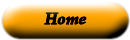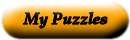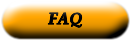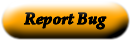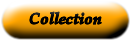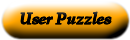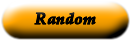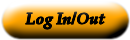### Grade 4 Math Power Words

Match the term to the correct definition.

 Math word match a straight path joining 2 points. the two points are called "endpoints" (------------------- ) hundredth a letter or other symbol that represents a number example 5 + X = 9 and x=4 to make the number sentence true intersect a figure measuring less than 90* that is formed by two rays or two line segments with a common endpoint right triangle a dot used to separate the ones and the tenths place in decimal numbers acute angle the digit two spaces to the right of a decimal can be written as 1/100 or 0.01 obtuse angle a number that is written using both a whole number and a fraction right angle crossing or meeting at right angles. The symbol means is perpendicular to endpoint one of two numbers that are multiplied to give a product angles common fractions that serve as benchmarks or points of reference to help us estimate other fractions. lines a triangle that has a right angle (90*) mixed number a line drawn through a figure that creates two "mirror" images of eachother perpendicular a whole number with more than two factors ray an amount left over when one number is divided by another number benchmark fractions to meet or cross common denominator a straight path that extends infinitely in opposite directions degree if two fractions have the same denominator decimal notation a straight path that starts at one point (the endpoint) and continues forever in one direction line segment a model that shows the amount of surface inside a closed boundary (such as a shape labeled in square units) line of symmetry a unit of measure for angles based on dividing up a circle into 360 parts. A unit of measure for temperature. parallel a figure measuring exactly 90* that is formed by two rays or two line segments with a common endpoint variable a whole number that has exactly two factors: itself and 1 factor [no clue] prime number a point at the end of a line segment or ray composite number ( ) lines, line segments, rays or ("planes) are said to be parallel if they never cross or meet, no matter how far they extend remainder a figure that is formed by two rays or two line segments with a common endpoint area model a figure measuring more that 90* and less than 180* that is formed by two rays or two line segments with a common endpoint

Use the "Printable HTML" button to get a clean page, in either HTML or PDF, that you can use your browser's print button to print. This page won't have buttons or ads, just your puzzle. The PDF format allows the web site to know how large a printer page is, and the fonts are scaled to fill the page. The PDF takes awhile to generate. Don't panic!Web armoredpenguin.com